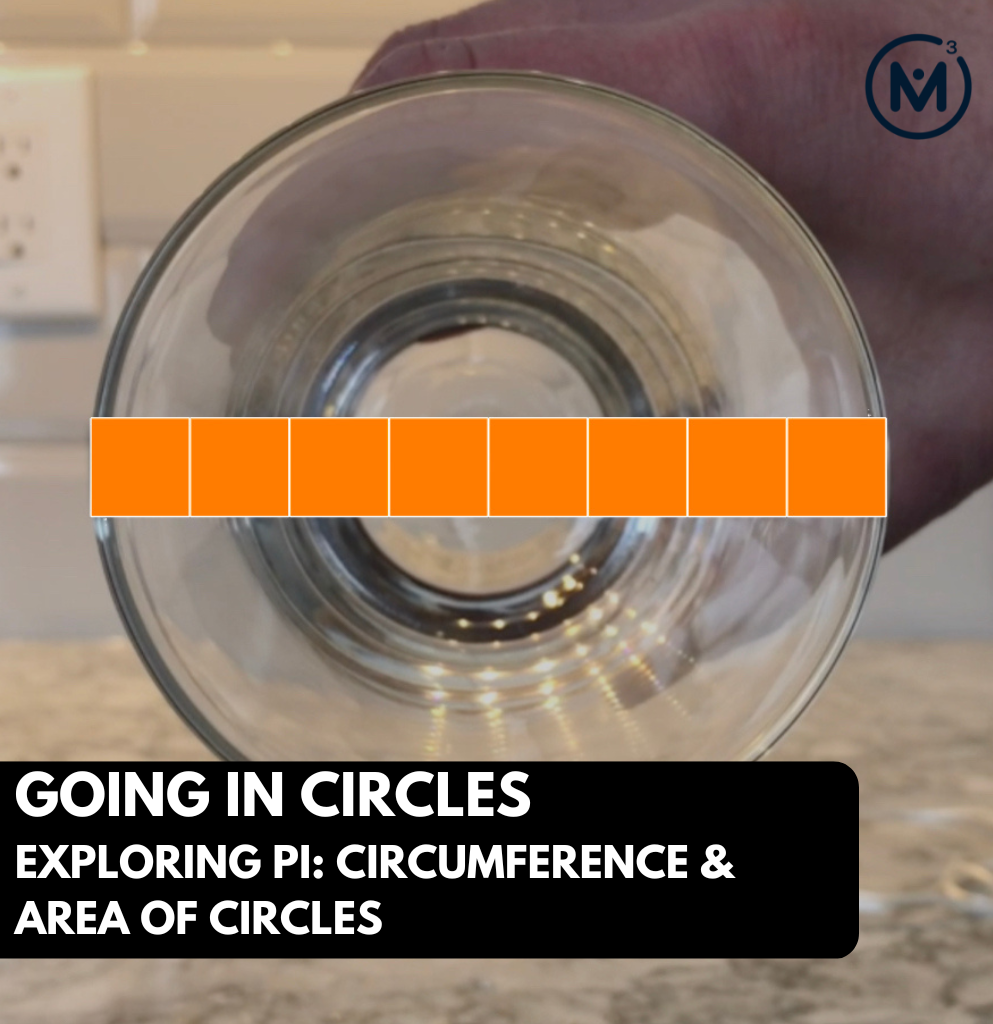## GOING IN CIRCLES [DAY 8]

### EXPLORING PI: CIRCUMFERENCE & AREA OF CIRCLES

Leverage contextual problems to investigate and emerge Pi to help conceptualize formulas for both the circumference and are of a circle.

## Intentionality & Unit Overview### Access each lesson from this unit using the navigation links below

Students will demonstrate the concepts and skills that they have developed through this context and learning experience related to circumference, diameter and area of a circle.

## Intentionality…

Through this purposeful practice, students will apply the concept of circumference and area of a circle to deepen understanding and begin building procedural fluency related to big ideas including the following:

• When exploring measurement relationships of any circle, there are three (3) standard measures that can be used to reveal more information about the circle:
• Circumference: the perimeter of a circle.
• Diameter: any straight line segment beginning and ending on the outer edge of a circle and passes through its centre.
• Radius: any straight line segment beginning at the centre of a circle and ending on the outer edge of the circle.
• Knowing one of the standard measures of a circle provides information about all other measures;
• There is a multiplicative relationship between the length of the diameter and the circumference of a circle. In other words, as the diameter of a circle increases, the circumference increases multiplicatively (not additively);
• For all circles, the ratio of the circumference to the diameter is the same (approximately 22:7); and,
• For all circles, the rate of each unit of circumference length per unit of diameter length is the same (approximately $$\frac{22}{7}$$ or 3.14) and is known as Pi, π.
• The area of a circle can be determined by decomposing and recomposing the figure into familiar polygons (i.e.: rectangles or parallelograms); and,
• For all circles, changing the diameter or radius will affect the area and circumference in predictable ways.

The independent assessment will allow students to demonstrate concepts and skills related to the following learning goals:

• I will use Pi (π) to determine an unknown length measurement when given the length of another measurement.
• I will use the formulas for determining the circumference of a circle and the area of a circle and explain how they work.
• I will apply my understanding of circumference and area of a circle to determine the perimeters and areas of composite figures.

Students might write and/or model their answers independently. Students can also be interviewed by their teacher.

An alternative assessment opportunity should be offered if this tool will not successfully capture what the student knows, understands or can do.

## String of Related Problems

Login/Join to access the entire Teacher Guide, downloadable slide decks and printable handouts for this lesson and all problem based units.

## Assessment Considerations…

Login/Join to access the entire Teacher Guide, downloadable slide decks and printable handouts for this lesson and all problem based units.

## Assessment: Pi (π), Circumference and Area of a Circle

Question #1:

Login/Join to access the entire Teacher Guide, downloadable slide decks and printable handouts for this lesson and all problem based units.

Question #2:

Login/Join to access the entire Teacher Guide, downloadable slide decks and printable handouts for this lesson and all problem based units.

Question #3:

Login/Join to access the entire Teacher Guide, downloadable slide decks and printable handouts for this lesson and all problem based units.

Question #4:

Login/Join to access the entire Teacher Guide, downloadable slide decks and printable handouts for this lesson and all problem based units.

Question #5:

Login/Join to access the entire Teacher Guide, downloadable slide decks and printable handouts for this lesson and all problem based units.

Login/Join to access the entire Teacher Guide, downloadable slide decks and printable handouts for this lesson and all problem based units.

## Educator Discussion Area

Login/Join to access the entire Teacher Guide, downloadable slide decks and printable handouts for this lesson and all problem based units.

## Explore Our 60+ Problem Based Units

This Make Math Moments Lesson was designed to spark curiosity for a multi-day unit of study with built in purposeful practice, number talks and extensions to elicit and emerge strategies and mathematical models.

Dig into our other units of study and view by concept continuum, grade or topic!